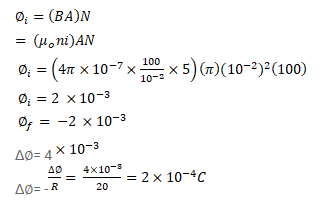# A long solenoid of radius 2 cm has 100 turns/cm

Question:

A long solenoid of radius $2 \mathrm{~cm}$ has 100 turns/cm and carries a current of $5 \mathrm{~A}$. A coil of radius $1 \mathrm{~cm}$ having 100 turns and a total resistance of $20 \Omega$ is placed inside the solenoid coaxially. The coil is connected to a galvanometer. If the current in the solenoid is reversed in direction, find the charge flown through the galvanometer.

Solution: